# NOTE: The following information is for Pure Compounds only. We are working on similar information for Binary Mixtures.

## Fitted Equations for Specific Properties

Listed below are the properties for which evaluated data is generated by TDE. Each property is linked to a list showing the set of possible equations that can be fit to the data. The equation that is used for a specific query is the one that provides the best fit of the data. This equation is then used to provide evaluated data.

NOTE: All of this information is displayed here in an individual file so that you can do text searches by using the Find funtion from the Edit menu of your browser.

Purpose: Provide definitions of equations and equation parameters used for output of evaluated data.

Select a property to see all associated equations. Properties represented with equations in TDE output are:

• Phase Boundary Pressure:
• Density (Phase Boundary):
• Density (Single Phase):
• Enthalpy of Phase Change:
• Heat Capacity:
• Speed of Sound (Single Phase):
• Refractive Index (Single Phase):
• Surface Tension:
• Viscosity (Single Phase):
• Thermal Conductivity :

## Wagner 25 (Vapor Pressure: Output Details)

ln(p/po) – ln(pc/po) = Tc/T (A1 + A2×t1.5 + A3×t2.5 + A4×t5); where t = 1 - T/Tc and po = 1 kPa

Evaluation Results: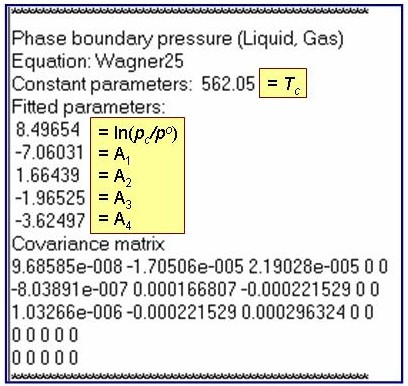The example is for fitted vapor pressures for benzene.

## Antoine (Vapor Pressure: Output Details)

ln(p/po) = A + B/(T + C); where po = 1 kPa

Evaluation Results: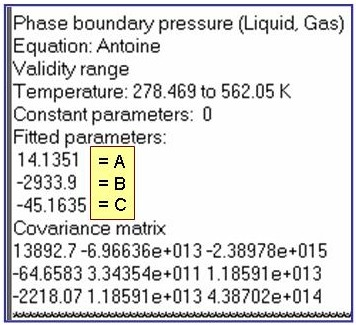The example is for fitted vapor pressures for benzene.

## Yaws (Vapor Pressure: Output Details)

lg(p/po) = a + b/T + c×lg(T) + d×T + e/T2 ; where po = 1 kPa and lg = log10

Evaluation Results: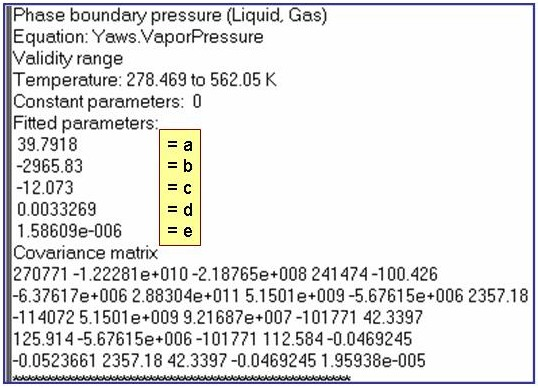The example is for fitted vapor pressures for benzene.

## DIPPR 115 (Vapor Pressure: Output Details)

ln(p/po) = a + b/T + c×ln(T) + d×T2 + e/T2 ; where po = 1 kPa

Evaluation Results:The example is for fitted vapor pressures for benzene.

## DIPPR 101 (Vapor Pressure: Output Details)

ln(p/po) = a + b/T + c×ln(T) + d×Te ; where po = 1 kPa

Evaluation Results: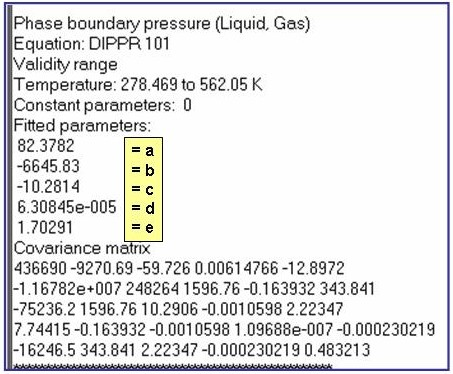The example is for fitted vapor pressures for benzene.

## Wagner36 (Vapor Pressure: Output Details)

ln(p/po) – ln(pc/po) = Tc/T (A1 + A2×t1.5 + A3×t3 + A4×t6); where t = 1 - T/Tc and po = 1 kPa

Evaluation Results: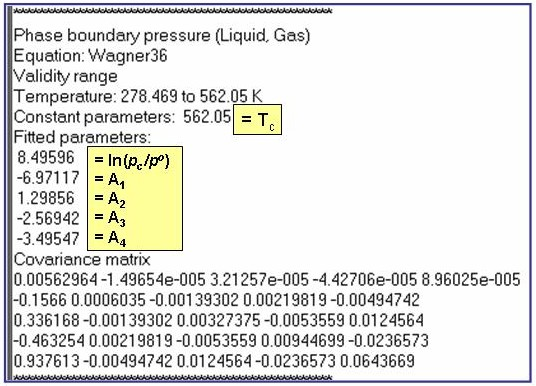The example is for fitted vapor pressures for benzene.

## PV Expansion: Sublimation Pressure

ln(p/po) = a1 + a2/T + a3×ln(T) + a4×T + a5×T2 + a6×T6+ a7/T4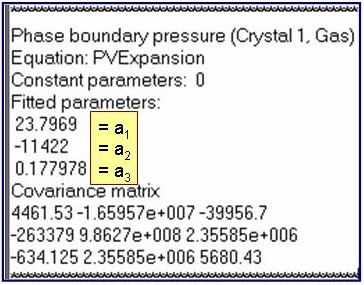NOTE: The TDE program truncates this equation based upon the extent and quality of the fitted data.

## Condensed Phase boundary pressure

• DEFAULT: PolynomialPressure: (sample parameter output)

ln(p/po) = å ai× T i, where the summation is from i = 0 to nTerms - 1

• Alternative 1:
There are no alternative equations for this property at this time.

## PolynomialExpansion: Phase boundary pressure for the crystal/liquid boundary

ln(p/po) = å ai× T i, where the summation is from i = 0 to nTerms - 1

Evaluation Results: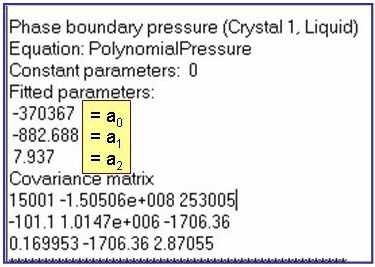The example is for fitted phase-boundary pressures for benzene.

## Density of the Liquid (on the Liquid/Gas phase boundary): dsat

• VDNS Expansion: (sample parameter output)

dsat = dc + a1×t0.35 + åai+1×ti , where the summation is from i = 1 to nTerms -1.

dc = the critical density and t = 1 - T/Tc, where Tc is the critical temperature.

• Alternative 1: Rackett (sample parameter output)
dsat = dc×B– (1-T/Tc)N; where Tc and dc are the critical temperature and critical density, respectively.
• Alternative 2: PPDS 10 (sample parameter output)
dsat = dc + a1×t0.35 + a2×t2/3 + a3×t + a4×t4/3;
dc = the critical density and t = 1 - T/Tc, where Tc is the critical temperature.
• Alternative 3: PPDS 17 (sample parameter output)
dsat = (a1 + a2×t) -1 - t-2/7/a0 ; where t = 1 - T/Tc, and Tc is the critical temperature.

## VDNS Expansion: For dsat of the Liquid or Gas phase

dsat = dc + a1×t0.35 + åai+1×ti ; where the summation is from i = 1 to nTerms -1.

dc = the critical density and t = 1 - T/Tc, where Tc is the critical temperature.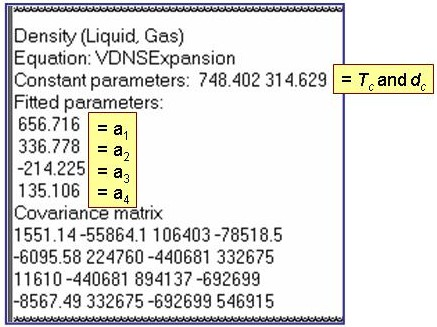NOTE: The TDE program truncates this equation based upon the extent and quality of the fitted data.

## Rackett Equation: For dsat of the Liquid phase

dsat = dc×B– (1-T/Tc)N; where Tc and dc are the critical temperature and critical density, respectively.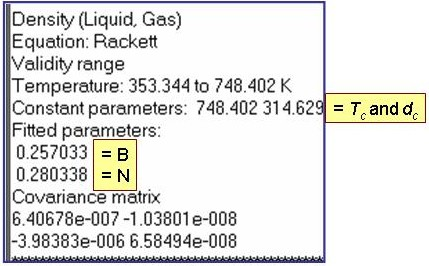## PPDS 10: For dsat of the Liquid phase

dsat = dc + a1×t0.35 + a2×t2/3 + a3×t + a4×t4/3;

dc = the critical density and t = 1 - T/Tc, where Tc is the critical temperature.

Evaluation Results: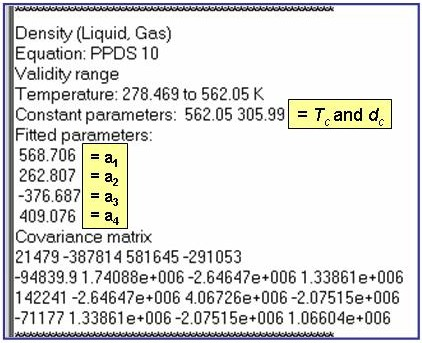The example is for fitted densities at vapor saturation for benzene.

## PPDS 17: For dsat of the Liquid phase

dsat = (a1 + a2×t) -1 - t-2/7/a0 ; where t = 1 - T/Tc, and Tc is the critical temperature.

Evaluation Results: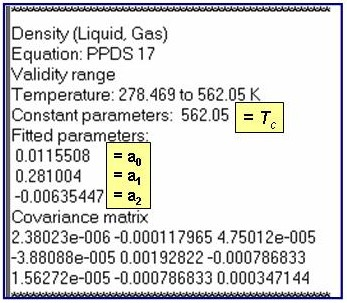The example is for fitted densities at vapor saturation for benzene.

## Density of the Gas (on the Liquid/Gas phase boundary)

• VDNSExpansion: (sample parameter output)

dsat = dc + a1×t0.35 + åai+1×ti , where the summation is from i = 1 to nTerms -1.

dc = the critical density and t = 1 - T/Tc, where Tc is the critical temperature.

• Alternative 1: There are no alternative equations for this property at this time.

## Density (Liquid Phase)

• Tait Equation: (sample parameter output)

d = dsat / [1 - c×ln{(b + p) / (b + psat)}] ; where

b = åBi+1×t i, c = åCi+1×t i, and t = (T-Tcenter)/100, where Tcenter is a constant parameter.
Summations are from i = 0 to (nTerms -1), where nTerms is the number of B or C terms.
dsat = the density of the saturated liquid and psat = the saturation vapor pressure.

• Alternative 1: There are no alternative equations for this property at this time.

## Tait Equation: Density (Liquid Phase)

d = dsat / [1 - c×ln{(b + p) / (b + psat)}] ; where

b = åBi+1×t i, c = åCi+1×t i, and t = (T-Tcenter)/100, where Tcenter is a constant parameter.
Summations are from i = 0 to (nTerms -1), where nTerms is the number of B or C terms.
dsat = the density of the saturated liquid and psat = the saturation vapor pressure.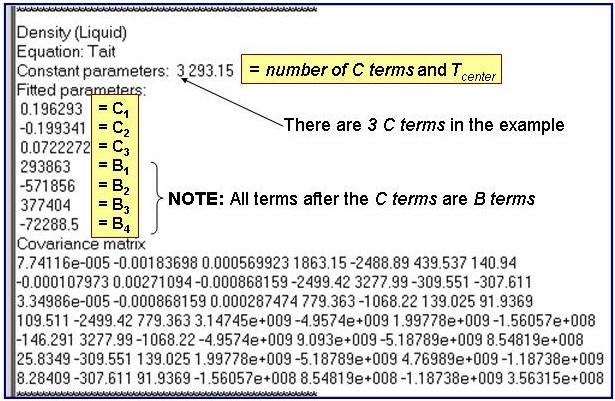## Density (Gas Phase)

• DEFAULT: Virial Equation (VirialV):
(sample parameter output)

d = 1000×Mw / Vm; where Mw is the molar mass and Vm is the molar volume.

Z = 1000×p×Vm/(R×T) = 1 + B/Vm + C/Vm2,

where B = åbi+1/T i and C = åci+1/T i

• Alternative 1:
There are no alternative equations for this property at this time.

## VirialV: For density of the gas phase.

d = 1000×Mw / Vm; where Mw is the molar mass and Vm is the molar volume.

Z = 1000×p×Vm/(R×T) = 1 + B/Vm + C/Vm2, where B = åbi+1/T i and C = åci+1/T i

Evaluation Results: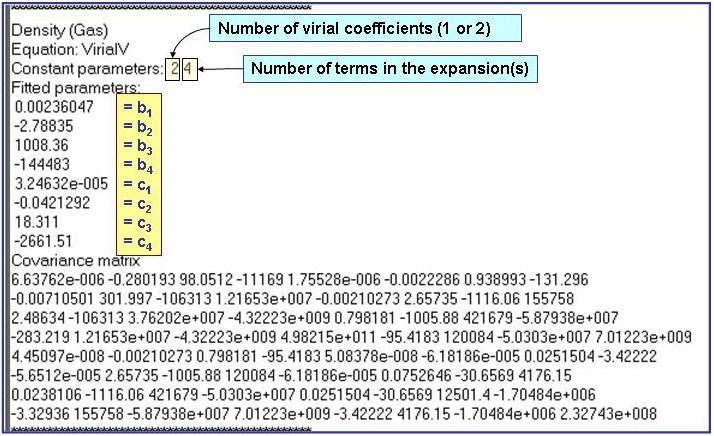The example is for fitted gas densities of pentane.

## Enthalpy of Vaporization (Liquid/Gas)

• DEFAULT: HVP Expansion: (sample parameter output)

ln(Hvap/Hvapo) = a1 + åai ×Tri-1×ln(1-Tr) , where the summation is from i = 2 to nTerms -1

Tr = T/Tc, Tc is the critical temperature, and Hvapo = 1 kJ/mol

• Alternative 1: Yaws.VaporizationH (sample parameter output)

Hvap = A×{1 - ( T / Tc )}n

• Alternative 2: PPDS 12 (sample parameter output)

Hvap/R = a1×t1/3 + a2×t2/3 + a3×t + a4×t2 + a5×t6

where t = 1 - T/Tc, Tc is the critical temperature, and R is the gas constant.

## HVPExpansion: For Hvap for the liquid-gas phase boundary

ln(Hvap/Hvapo) = a1 + åai ×Tri-1×ln(1-Tr) , where the summation is from i = 2 to nTerms -1

Tr = T/Tc, Tc is the critical temperature, and Hvapo = 1 kJ/mol

Evaluation Results: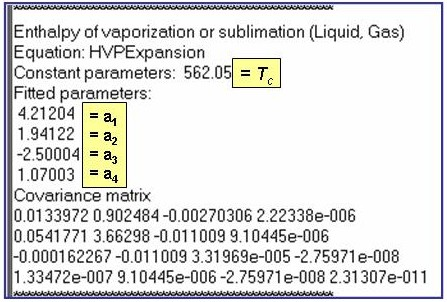The example is for fitted enthalpies of vaporization for benzene.

## Yaws.VaporizationH: For Hvap for the liquid-gas phase boundary

Hvap = A×{1 - ( T / Tc )}n

Evaluation Results: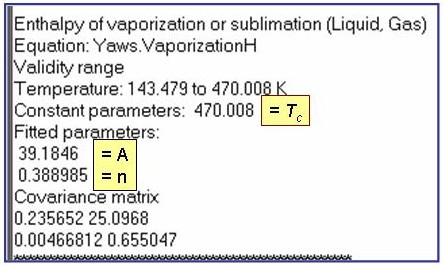The example is for fitted enthalpies of vaporization for pentane.

## PPDS12: For Hvap for the liquid-gas phase boundary

Hvap/R = a1×t1/3 + a2×t2/3 + a3×t + a4×t2 + a5×t6

where t = 1 - T/Tc, Tc is the critical temperature, and R is the gas constant.

Evaluation Results: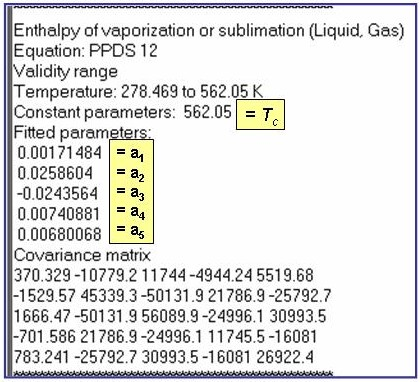The example is for fitted enthalpies of vaporization for benzene.

## Csat(Liquid Phase)

• DEFAULT: CSExpansion: (sample parameter output)

Csat = (åai+1× ti) + b/t , where the summation is from i = 0 to nTerms -1.

• Alternative 2: Yaws.PolynomialExpansion (sample parameter output)

Csat = å ai× T i, where the summation is from i = 0 to nTerms - 1

• Alternative 3: DIPPR.PolynomialExpansion (sample parameter output)

Csat = å ai× T i, where the summation is from i = 0 to nTerms - 1

• Alternative 3: PPDS 15 (sample parameter output)

Csat / R = a0/t + a1 + a2×t + a3×t2 + a4×t3 + a5×t4

where t = 1 - T/Tc, Tc is the critical temperature, and R is the gas constant.

## CSExpansion: For Csat of the Liquid phase

Csat = (åai+1× ti) + b/t , where the summation is from i = 0 to nTerms -1.

Evaluation Results: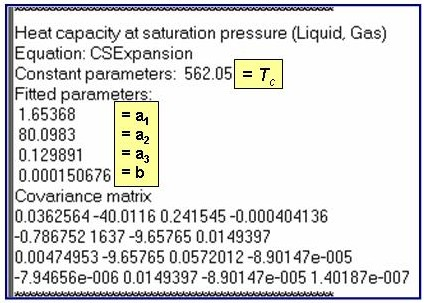The example is for fitted heat capacities at vapor saturation for benzene.

## Yaws.PolynomialExpansion: For Csat of the liquid phase

Csat = å ai× T i, where the summation is from i = 0 to nTerms - 1

Evaluation Results: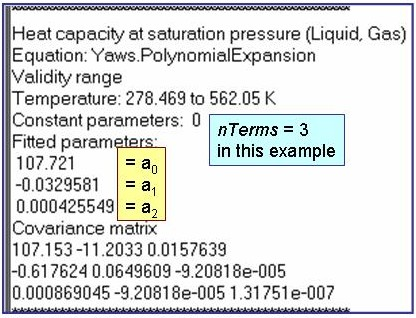The example is for fitted heat capacities at vapor saturation for benzene.

## DIPPR.PolynomialExpansion: For Csat of the liquid phase

Csat = å ai× T i, where the summation is from i = 0 to nTerms - 1

Evaluation Results: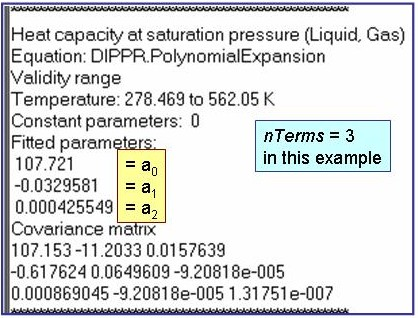The example is for fitted heat capacities at vapor saturation for benzene.

## PPDS 15: For Csat of the liquid phase

Csat / R = a0/t + a1 + a2×t + a3×t2 + a4×t3 + a5×t4

where t = 1 - T/Tc, Tc is the critical temperature, and R is the gas constant.

Evaluation Results: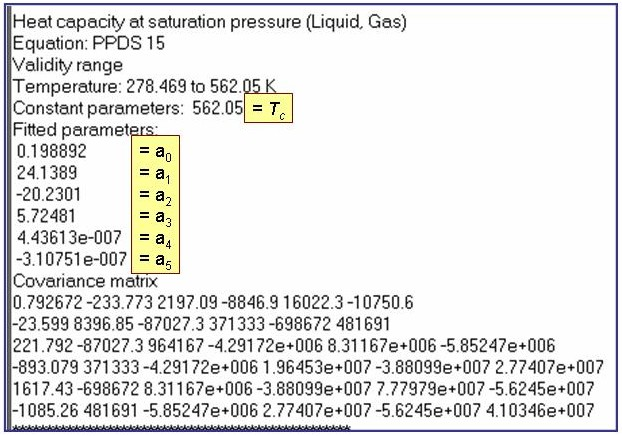The example is for fitted heat capacities at vapor saturation for benzene.

## Cpo(Ideal Gas)

• DEFAULT: Wilhoit Equation

Cpo / R = a0 + (a1 /T 2) × exp( -a2 /T ) + a3 × y2 + {a4 - a5 /(T - a7)2} × y 8

where    y = (T - a7)/(T + a6)    and R is the gas constant.

See Thermodynamics of Organic Compounds in the Gas State (Volumes I and II) by M. Frenkel, G. J. Kabo, K. N. Marsh, G. N. Roganov, and R. C. Wilhoit. Publsihed by the Thermodynamics Research Center (TRC), College Station: TX. 1994.

• Alternative 1: Yaws.PolynomialExpansion (sample parameter output)

Cpo = å ai× T i, where the summation is from i = 0 to nTerms - 1

• Alternative 2: AlyLee (which is also DIPPR 107) (sample parameter output)

Cpo = a + b×{(c/T)/sinh(c/T)}2 + d×{(e/T)/cosh(e/T)}2

• Alternative 3: PPDS2 (sample parameter output)

Cpo/R = Clow + (Clow - C¥)×y2 ×{1 + (y - 1) å(ai × yi)} ; where the summation is from i = 0 to 4.

Clow and C¥ are equation constants, and y = T / (T + TS), where TS is a constant.

• Alternative 4: Helmholtz (sample parameter output)

Cpo/R = 1 + t - {åai×ni×(ni - 1)×tni}(ni¹ 0 or 1) + åbi×(ci×t)2×exp(ci×t) / {exp(ci×t) - 1}2;

where t = Tc/T, Tc = the critical temperature, and the summations are from i = 1 to nTerms.

## Cpo(Ideal Gas)

Cpo = å ai× T i, where the summation is from i = 0 to nTerms - 1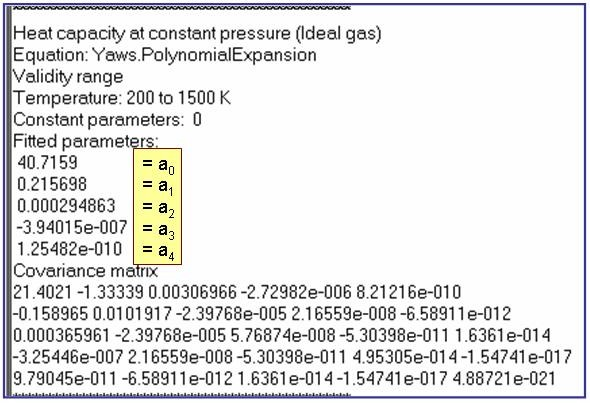The example is for fitted heat capacities in the ideal-gas state at p = 100 kPa for pentane.

## Aly & Lee Equation (same as DIPPR 107):Cpo(Ideal Gas)

Cpo = a + b×{(c/T)/sinh(c/T)}2 + d×{(e/T)/cosh(e/T)}2

Reference: Aly, F. A.; Lee, L. L. Fluid Phase Equil. 1981, 6, 169-179.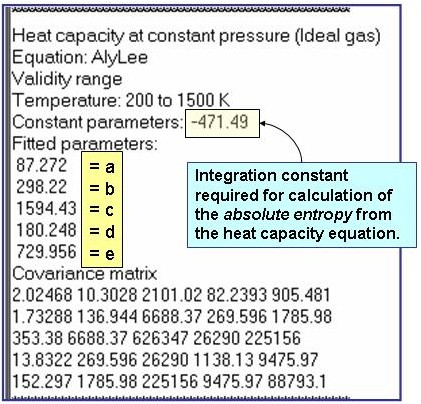The example is for fitted heat capacities in the ideal-gas state at p = 100 kPa for pentane.

## PPDS 2: Cpo(Ideal Gas)

Cpo/R = Clow + (Clow - C¥)×y2 ×{1 + (y - 1) å(ai × yi)} ; where the summation is from i = 0 to 4.

Clow and C¥ are equation constants, and y = T / (T + TS), where TS is a constant.

Evaluation Results: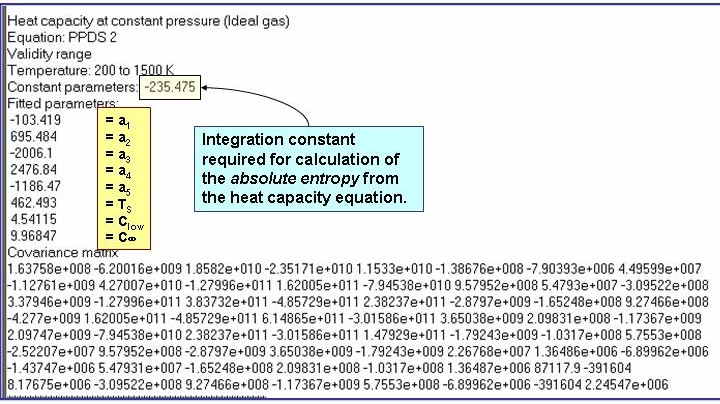The example is for fitted heat capacities in the ideal-gas state at p = 100 kPa for pentane.

## Helmholtz: Cpo(Ideal Gas)

Cpo/R = 1 + t - {åai×ni×(ni - 1)×tni}(ni¹ 0 or 1) + åbi×(ci×t)2×exp(ci×t) / {exp(ci×t) - 1}2;
where t = Tc/T, Tc = the critical temperature, and the summations are from i = 1 to nTerms.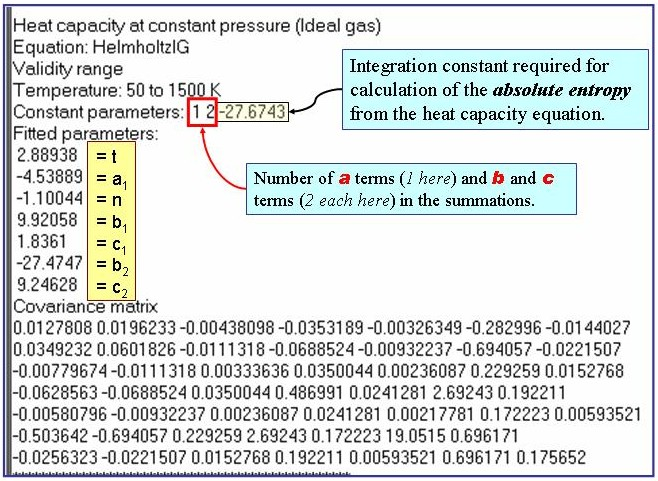The example is for fitted heat capacities in the ideal-gas state at p = 100 kPa for 2-methylpyrrole.

## Speed of Sound (Liquid Phase)

• DEFAULT: RSSL Expansion: (sample parameter output)

u = A + B×T + C×(d - dsat) + D×d 2,

where d is the density of the liquid and dsat is the density of the saturated liquid.

• Alternative 1:
There are no alternative representations for this property at this time.

## Speed of Sound (Liquid Phase)

u = A + B×T + C×(d - dsat) + D×d 2,

where d is the density of the liquid and dsat is the density of the saturated liquid.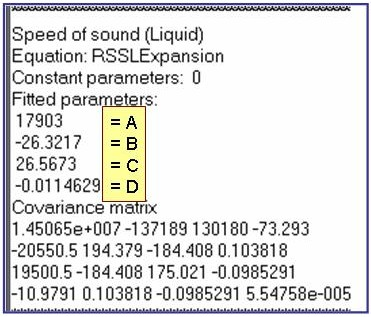The example is for fitted speeds of sound for benzene.

## Speed of Sound (Gas Phase)

• DEFAULT: RSSG Expansion: (sample parameter output)

u = A + B×T + C×p + D×p/T ;

where T is the temperature and p is pressure.

• Alternative 1:
There are no alternative representations for this property at this time.

## Speed of Sound (Gas Phase)

u = A + B×T + C×p + D×p/T ;

where T is the temperature and p is pressure.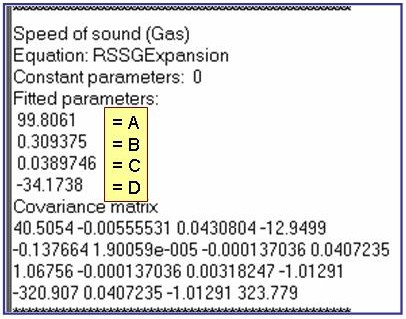The example is for fitted speeds of sound for pentane in the gas phase.

## Refractive Index nD(Liquid)

• DEFAULT: RIXExpansion: (sample parameter output)

nD = A + B×t + å Ci×wi ;the summation is from i = 1 to nTerms

where t = T - 298.15 K and w = WL - 589.26 (WL = wavelength in nm)

• Alternative 1:
There are no alternative equations for this property at this time.

## RIXExpansion: Refractive Index nD(Liquid)

nD = A + B×t + å Ci×wi ;the summation is from i = 1 to nTerms

where t = T - 298.15 K and w = WL - 589.26 (WL = wavelength in nm)

Evaluation Results: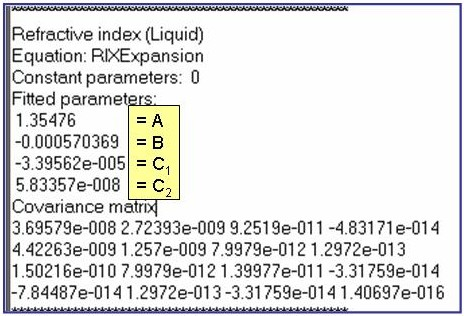The example is for fitted surface tensions for pentane.

## Surface Tension s(Liquid/Gas)

• DEFAULT: PPDS 14: (sample parameter output)

s = a0× ta1×(1 + a2 ×t) , where t = 1 - T/Tc and Tc is the critical temperature.

• Alternative 1: Yaws.SurfaceTension (sample parameter output)

s = exp(A)×{1 - ( T / Tc )}n

• Alternative 2: HVPExpansion (sample parameter output)

ln(s/so) = a1 + åai ×Tri-1×ln(1-Tr) , where the summation is from i = 2 to nTerms -1

Tr = T/Tc, Tc is the critical temperature, and so = 1 N/m

## PPDS14: For surface tension s for the liquid/gas interface

s = a0× ta1×(1 + a2 ×t) , where t = 1 - T/Tc and Tc is the critical temperature.

Evaluation Results: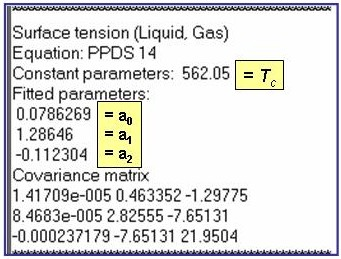The example is for fitted surface tensions for benzene.

## Yaws.SurfaceTension: For surface tension s for the liquid/gas interface

• Alternative 1: Yaws.SurfaceTension (sample parameter output)

s = exp(A)×{1 - ( T / Tc )}n

Evaluation Results: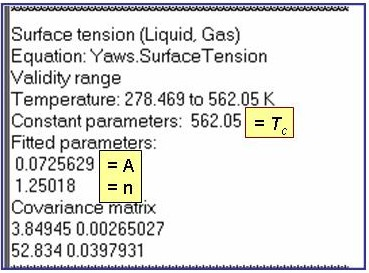The example is for fitted surface tensions for benzene.

## HVPExpansion: For surface tension s for the liquid/gas interface

ln(s/so) = a1 + åai ×Tri-1×ln(1-Tr) , where the summation is from i = 2 to nTerms -1

Tr = T/Tc, Tc is the critical temperature, and so = 1 N/m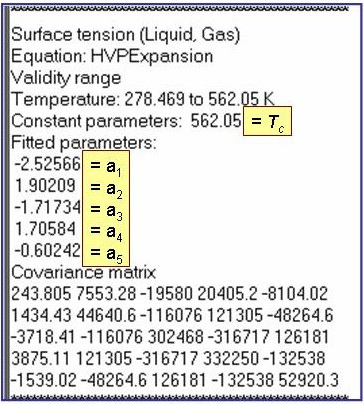The example is for fitted surface tensions for benzene.

## PPDS9: Viscosity h of the saturated liquid

ln(h/ho) = a1 ×X1/3 + a2 ×X4/3 + ln(a5) , where X = (a3 - T) / (T - a4) and ho = 1 Pa×s.

Evaluation Results: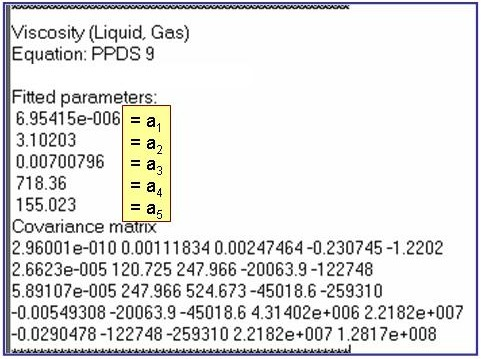The example is for fitted viscosities for the saturated liquid phase of benzene.

## Yaws.Viscosity: Viscosity h of the saturated liquid

lg(h/ho) = A + B/T + C×T + D×T 2

Evaluation Results: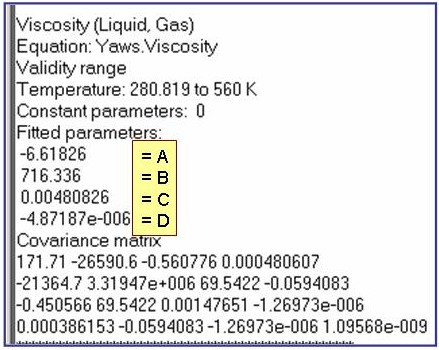The example is for fitted viscosities for the saturated liquid phase of benzene.

## DIPPR101: Viscosity h of the saturated liquid

ln(h/ho) = a + b/T + c×ln(T) + d×Te ; where ho = 1 Pa×s

Evaluation Results: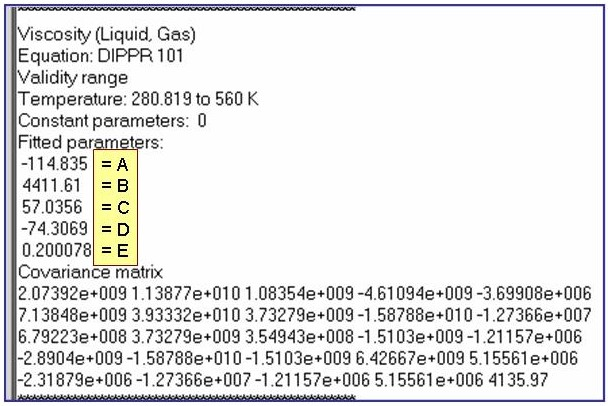The example is for fitted viscosities for the saturated liquid phase of benzene.

## TransportPolynomial: For viscosity h of the gas at low pressures (p < 600 kPa)

h = å ai× T i, where the summation is from i = 0 to nTerms - 1

Evaluation Results: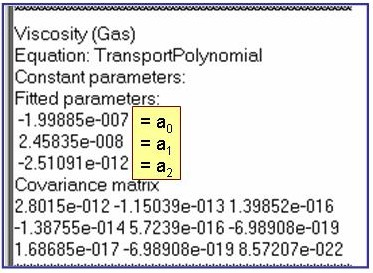The example is for fitted thermal conductivities for the saturated liquid phase of pentane.

## Yaws.PolynomialExpansion: For viscosity h of the gas at low pressures (p < 600 kPa)

h = å ai× T i, where the summation is from i = 0 to nTerms - 1

Evaluation Results: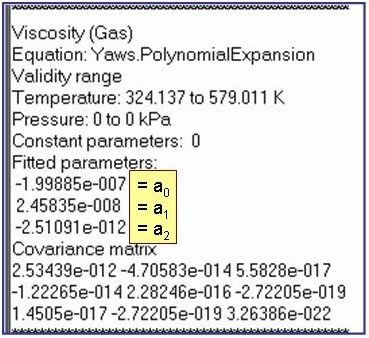The example is for fitted thermal conductivities for the saturated liquid phase of pentane.

## DIPPR 102: For viscosity h of the gas at low pressures (p < 600 kPa)

h = A×T B / (1+ C/T + D/T 2)

Evaluation Results: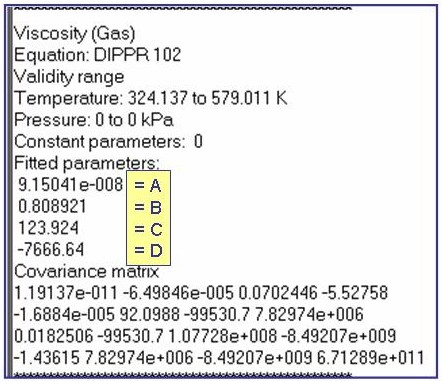The example is for fitted thermal conductivities for the saturated liquid phase of pentane.

## PPDS 5: For viscosity h of the gas at low pressures (p < 600 kPa)

h = a0×Tr / {1 + a1×(Tr - 1)× Tra2}1/6; where Tr = T/Tc and Tc is the critical temperature.

Evaluation Results: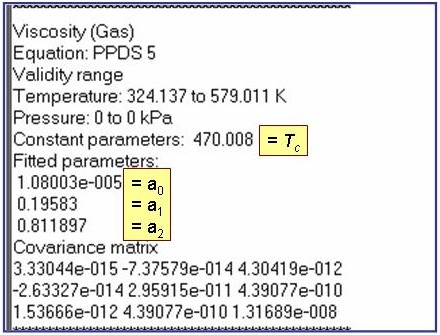The example is for fitted thermal conductivities for the saturated liquid phase of pentane.

## TransportPolynomial: For thermal conductivity l of the saturated liquid

l = å ai× T i, where the summation is from i = 0 to nTerms - 1

Evaluation Results: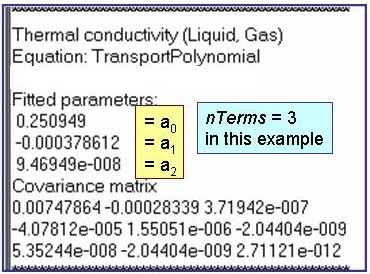The example is for fitted thermal conductivities for the saturated liquid phase of benzene.

## Yaws.ThermalConductivity: For thermal conductivity l of the saturated liquid

lg(l/lo) = A + B×(1 - T/C)2/7

• Evaluation Results: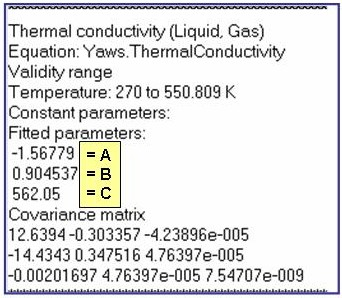The example is for fitted thermal conductivities for the saturated liquid phase of benzene.

## Yaws.PolynomialExpansion: For thermal conductivity l of the saturated liquid

l = å ai× T i, where the summation is from i = 0 to nTerms - 1

Evaluation Results: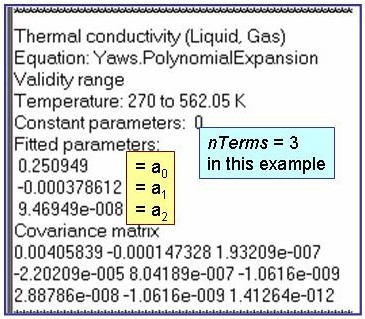The example is for fitted thermal conductivities for the saturated liquid phase of benzene.

## DIPPR.PolynomialExpansion: For thermal conductivity l of the saturated liquid

l = å ai× T i, where the summation is from i = 0 to nTerms - 1

Evaluation Results: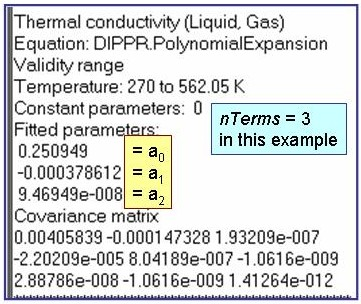The example is for fitted thermal conductivities for the saturated liquid phase of benzene.

## PPDS8: For thermal conductivity l of the saturated liquid

l = a1 + å(ai+1 ×ti/3) , where the summation is from i = 1 to nTerms -1 and t = 1 - T/Tc

Evaluation Results: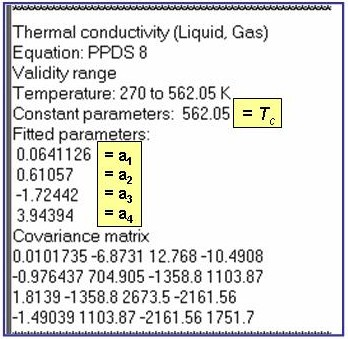The example is for fitted thermal conductivities for the liquid phase of benzene.

## Thermal Conductivity (Gas at low pressures; p < 6 bar)

• DEFAULT: TransportPolynomial: (sample parameter output)

l = å ai× T i, where the summation is from i = 0 to nTerms - 1

• Alternative 1: Yaws.PolynomialExpansion (sample parameter output)

l = å ai× T i, where the summation is from i = 0 to nTerms - 1

• Alternative 2: PPDS 3 (sample parameter output)

l = Tr0.5 (åai / Tri)-1 , where the summation is from i = 1 to 3,

and Tr = T/Tc, where Tc is the critical temperature.

## TransportPolynomial: For thermal conductivity l of the gas at low pressure (p < 600 kPa)

l = å ai× T i, where the summation is from i = 0 to nTerms - 1

Evaluation Results: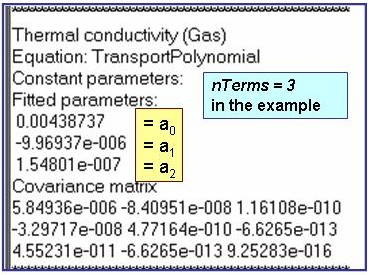The example is for fitted thermal conductivities for the saturated liquid phase of pentane.

## Yaws.PolynomialExpansion: For thermal conductivity l of the gas at low pressure (p < 600 kPa)

l = å ai× T i, where the summation is from i = 0 to nTerms - 1

Evaluation Results: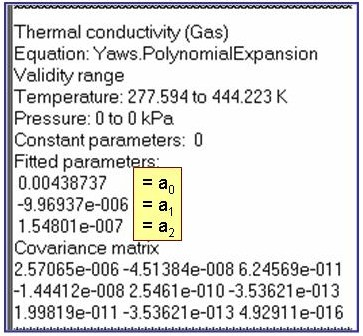The example is for fitted thermal conductivities for the saturated liquid phase of pentane.

## PPDS 3: For thermal conductivity l of the gas at low pressure (p < 600 kPa)

l = Tr0.5 (åai / Tri)-1 , where the summation is from i = 1 to 3,

and Tr = T/Tc, where Tc is the critical temperature.

Evaluation Results: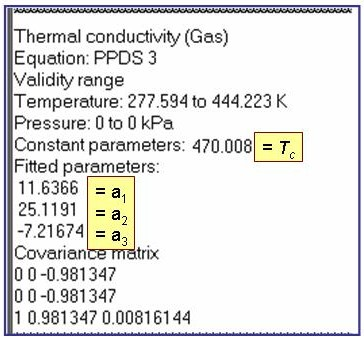The example is for fitted thermal conductivities for the saturated liquid phase of pentane.